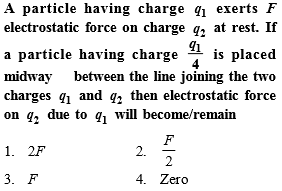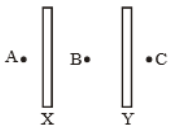Concept Questions :-

Coulomb's law
High Yielding Test Series + Question Bank - NEET 2020

Difficulty Level:

A charge q is to be divided on two small conducting spheres. What should be the value of charges on the spheres so that when placed at a certain distance apart, the repulsion force between them is maximum

(1)

(2)

(3)

(4)

Concept Questions :-

Coulomb's law
High Yielding Test Series + Question Bank - NEET 2020

Difficulty Level:

You are traveling in a car during a thunderstorm. In order to protect yourself from lightning, you would prefer to

(1) Touch the nearest electric pole

(2) Take shelter under a tree

(3) Get out and crouch on the ground

(4) Remain in the car

Concept Questions :-

Electric field
High Yielding Test Series + Question Bank - NEET 2020

Difficulty Level:

If a soap bubble is given some charge, then its radius

(1) Increases

(2) Decreases

(3) Remain unchanged

(4) May increase or decrease depending upon the give charge is positive or negative

Concept Questions :-

Coulomb's law
High Yielding Test Series + Question Bank - NEET 2020

Difficulty Level:

An electric dipole of dipole moment p is placed in an electric field of intensity E such that angle between electric field and dipole moment is $\theta$. Assuming that the potential energy of the dipole is zero when $\theta =0°$, the potential energy of the dipole will be

(1) -pE cos$\theta$

(2) pE(1-cos$\theta$)

(3) pE(cos$\theta$-1)

(4) -2pE(cos$\theta$-1)

Concept Questions :-

Electric dipole
High Yielding Test Series + Question Bank - NEET 2020

Difficulty Level:

A point charge q is placed over a horizontal square of side L at a normal distance of L/4 from its centre. Electric flux through the square is

1. $\mathrm{\varphi }=\frac{\mathrm{q}}{6{\mathrm{\epsilon }}_{0}}$

2. $\mathrm{\varphi }<\frac{\mathrm{q}}{6{\mathrm{\epsilon }}_{0}}$

3. $\frac{\mathrm{q}}{6{\mathrm{\epsilon }}_{0}}<\mathrm{\varphi }<\frac{\mathrm{q}}{{\mathrm{\epsilon }}_{0}}$

4. $\mathrm{\varphi }>\frac{\mathrm{q}}{6{\mathrm{\epsilon }}_{0}}$

Concept Questions :-

Gauss law
High Yielding Test Series + Question Bank - NEET 2020

Difficulty Level:

What is the angle between the electric dipole moment and the electric field strength due to it on the equatorial line

(1) $0°$

(2) $90°$

(3) $180°$

(4) None of these

Concept Questions :-

Electric dipole

Difficulty Level:

The electrostatic field due to a charged conductor just outside the conductor is

1. zero and parallel to the surface at every point inside the conductor

2. zero and is normal to the surface at every point inside the conductor

3. parallel to the surface at every point and zero inside the conductor

4. normal to the surface at every point and zero inside the conductor

Concept Questions :-

Electric field
High Yielding Test Series + Question Bank - NEET 2020

Difficulty Level:

X and Y are large, parallel conducting plates close to each other. Each face has an area A. X is given a charge Q. Y is without any charge. Point A, B, and C are as shown in the figure. Find the incorrect option.1.  the field at B is $\frac{\mathrm{Q}}{2{\mathrm{\epsilon }}_{0}\mathrm{A}}$

2.  the field at B is $\frac{\mathrm{Q}}{{\mathrm{\epsilon }}_{0}\mathrm{A}}$

3.  the fields at A, B and C are of the same magnitude

4.  the fields at A and C are of the same magnitude but in opposite directions

Concept Questions :-

Electric field
High Yielding Test Series + Question Bank - NEET 2020

Difficulty Level:

A particle of mass m and charge q is at rest. If it is moved with velocity $\frac{\sqrt{3}c}{2}$ where c is speed of light in vacuum, then its charge will become/remain

(1) 2q

(2) $\sqrt{3}q$

(3) $\frac{q}{2}$

(4) q

Concept Questions :-

Ways of charging